# The Elf C0de⚓︎

Elf: Ribb Bonbowford
Objective: Operate the Santavator

## Request⚓︎

Ribb Bonbowford

Hello - my name is Ribb Bonbowford. Nice to meet you!
Are you new to programming? It's a handy skill for anyone in cyber security.
This challenge centers around JavaScript. Take a look at this intro and see how far it gets you!
Ready to move beyond `elf` commands? Don't be afraid to mix in native JavaScript.
Trying to extract only numbers from an array? Have you tried to `filter`?
Maybe you need to enumerate an object's keys and then filter?
Getting hung up on number of lines? Maybe try to minify your code.
Is there a way to `push` array items to the beginning of an array? Hmm...

## Hints⚓︎

`var array = [2, 3, 4]; array.push(1)` doesn't do QUITE what was intended...

Getting a Key Name

In JavaScript you can enumerate an object's keys using `keys`, and filter the array using `filter`.

Filtering Items
Compressing JS

There are lots of ways to make your code shorter, but the number of elf commands is key.

JavaScript Primer

Want to learn a useful language? JavaScript is a great place to start! You can also test out your code using a JavaScript playground.

JavaScript Loops

Did you try the JavaScript primer? There's a great section on looping.

## Solution⚓︎

Only the first 6 levels count towards solving the challenge. Bonus levels 7 and 8 are not required (but a lot of fun). Some of the scripts include additional empty lines to enhance readability, but all solutions still fit within the maximum line requirements.

### Main levels

Level 1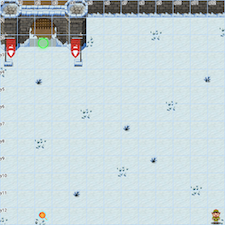```1 2``` ``````elf.moveLeft(10) elf.moveUp(10) ``````

Level 2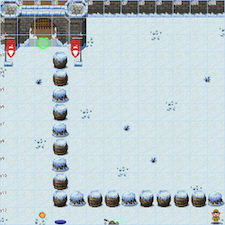```1 2 3 4 5``` ``````elf.moveLeft(6) var sum = elf.get_lever(0) + 2 elf.pull_lever(sum) elf.moveLeft(4) elf.moveUp(10) ``````

Level 3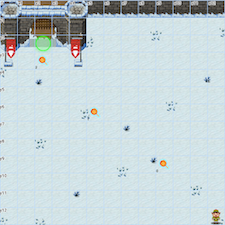```1 2 3 4``` ``````for (var i = 0; i < lollipop.length; i++) elf.moveTo(lollipop[i]); elf.moveUp(1) ``````

Level 4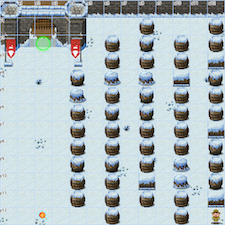```1 2 3 4 5 6``` ``````for (var i = 0; i < 3; i++) { elf.moveLeft(3) elf.moveUp(12) elf.moveLeft(3) elf.moveDown(12) } ``````

Level 5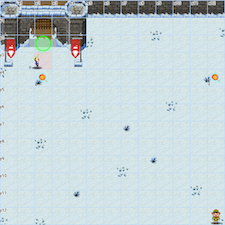``` 1 2 3 4 5 6 7 8 9 10``` ``````elf.moveTo(lollipop) elf.moveTo(munchkin) var data = elf.ask_munch(0) var newdata = [] for (var i = 0; i < data.length; i++) if (typeof data[i] === 'number') newdata.push(data[i]) elf.tell_munch(newdata) elf.moveUp(2) ``````

Level 6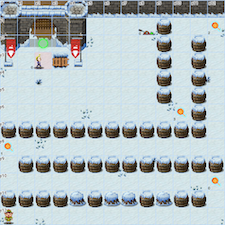``` 1 2 3 4 5 6 7 8 9 10 11 12 13 14``` ``````for (var i = 0; i < 4; i++) elf.moveTo(lollipop[i]) elf.moveTo(munchkin) var data = elf.ask_munch(0) var thekey = '' Object.keys(data).forEach(function(key) { if (data[key] === "lollipop") thekey = key }) elf.tell_munch(thekey) elf.moveUp(2) ``````

### Bonus levels

Level 7``` 1 2 3 4 5 6 7 8 9 10 11 12 13 14 15 16 17 18 19``` ``````function MyFilter(matrix) { var sum = 0 for(var i = 0; i < matrix.length; i++) for(var j = 0; j < matrix[i].length; j++) if (typeof matrix[i][j] === 'number') sum += matrix[i][j] return sum } var funcs = [elf.moveDown, elf.moveLeft, elf.moveUp, elf.moveRight] for (var i = 1; i <= 8; i++) { funcs[(i-1)%4](i) elf.pull_lever(i-1) } elf.moveUp(2) elf.moveLeft(4) elf.tell_munch(MyFilter) elf.moveUp(2) ``````

Level 8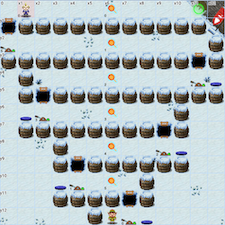``` 1 2 3 4 5 6 7 8 9 10 11 12 13 14 15 16 17 18 19 20 21 22 23 24``` ``````function MyFilter(matrix) { var sum = 0 var thekey = '' for(var i = 0; i < matrix.length; i++) Object.keys(matrix[i]).forEach(function(key) { if (matrix[i][key] === "lollipop") thekey = key }) return thekey } var lever_sum = 0 var hor_steps = 1 var funcs = [elf.moveRight, elf.moveLeft] for (var i = 0; i < 6; i++) { funcs[(i)%2](hor_steps) hor_steps += 2 lever_sum += elf.get_lever(i) elf.pull_lever(lever_sum) elf.moveUp(2) } elf.tell_munch(MyFilter) elf.moveRight(12) ``````

## Response⚓︎

Ribb Bonbowford

Wow - are you a JavaScript developer? Great work!
Hey, you know, you might use your JavaScript and HTTP manipulation skills to take a crack at bypassing the Santavator's S4.